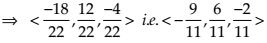Saturday, July 11, 2020
Home > CBSE Class 12 > NCERT Solutions Class 12 Maths Three Dimensional Geometry Exercise 11.1

# NCERT Solutions Class 12 Maths Three Dimensional Geometry Exercise 11.1# NCERT Solutions for Class 12 Maths Three Dimensional Geometry

Hi Students, Welcome to Amans Maths Blogs (AMB). In this post, you will get the NCERT Solutions for Class 12 Maths Three Dimensional Geometry Exercise 11.1.

As we know that all the schools affiliated from CBSE follow the NCERT books for all subjects. You can check the CBSE NCERT Syllabus. Thus, NCERT Solutions helps the students to solve the exercise questions as given in NCERT Books.

NCERT Solutions for class 12 is highly recommended by the experienced teacher for students who are going to appear in CBSE Class 12 and JEE Mains and Advanced and NEET level exams. Here You will get NCERT Solutions for Class 12 Maths 3D Geometry Exercise 11.1 of all questions given in NCERT textbooks of class 12 in details with step by step process.

The PDF books of NCERT Solutions for Class 12 are the first step towards the learning and understanding the each sections of Maths, Physics, Chemistry, Biology as it all help in engineering medical entrance exams. To solve it, you just need to click on download links of NCERT solutions for class 12.

CBSE Class 12th is an important school class in your life as you take some serious decision about your career. And out of all subjects, Maths is an important and core subjects. So CBSE NCERT Solutions for Class 12th Maths is major role in your exam preparation as it has detailed chapter wise solutions for all exercise. This NCERT Solutions can be downloaded in PDF file. The downloading link is given at last.

NCERT Solutions for Class 12 Maths are not only the solutions of Maths exercise but it builds your foundation of other important subjects. Getting knowledge of depth concept of CBSE Class 12th Maths like Algebra, Calculus, Trigonometry, Coordinate Geometry help you to understand the concept of Physics and Physical Chemistry.

## NCERT Solutions for Class 12 Maths Three Dimensional Geometry

NCERT Solutions for Class 12 Maths Three Dimensional Geometry Exercise 11.1 Ques No 1.

If a line makes angles 90°, 135°, 45° with the x, y and z-axes respectively, find its direction cosines

NCERT Solutions:

If a line makes angles α, β and γ with x, y and z-axes, then direction cosines are l = cos α, m = cos β and n = cos γ.

Given that α = 90°, β = 135° and γ = 45°. Thus, the direction cosines of the given line is

l = cos 90°, m = cos 135° and n = cos 45°.

or, l = 0, m = -1/√2, n = 1/√2.

NCERT Solutions for Class 12 Maths Three Dimensional Geometry Exercise 11.1 Ques No 2.

Find the direction cosines of a line which makes equal angles with the coordinate axes.

NCERT Solutions:

If a line makes angles α, β and γ with x, y and z-axes, then direction cosines are l = cos α, m = cos β and n = cos γ.

And, l2 + m2 + n2 = 1    …(1)

Given that α = β = γ. Thus, the direction cosines of the given line is

l = cos α, m = cos α and n = cos α.

or, l = m = n

or, l2 + l2 + l2 = 1

or, 3l2 = 1

or, l = ±1/√3

Thus, l = m = n = ±1/√3.

Therefore, the direction cosines of the given line are (1/√3, 1/√3, 1/√3) or (-1/√3, -1/√3, -1/√3).

NCERT Solutions for Class 12 Maths Three Dimensional Geometry Exercise 11.1 Ques No 3.

If a line has the direction ratios –18, 12, –4, then what are its direction cosines ?

NCERT Solutions:

Let a, b, c be direction ratios of a line, then the direction cosines of the line areGiven that the direction ratios of the line is a = –18, b = 12, c = –4. Then,Thus, the direction cosines of the line areNCERT Solutions for Class 12 Maths Three Dimensional Geometry Exercise 11.1 Ques No 4.

Show that the points (2, 3, 4), (– 1, – 2, 1), (5, 8, 7) are collinear.

NCERT Solutions:

The direction ratios of line joining A and B are (– 1 – 2), (– 2 – 3), (1 – 4) Or, – 3, – 5, –3.

The direction ratios of line joining B and C are (5 + 1), (8 + 2), (7 – 1) Or, 6, 10, 6

It is clear that direction ratios of AB and BC are proportional.

Hence, AB is parallel to BC.

But point B is common to both AB and BC. Therefore, A, B, C are collinear points.

NCERT Solutions for Class 12 Maths Three Dimensional Geometry Exercise 11.1 Ques No 5.

Find the direction cosines of the sides of the triangle whose vertices are (3, 5, – 4), (– 1, 1, 2) and (– 5, – 5, – 2).

NCERT Solutions:

error: Content is protected !!